Select Page

# Statistical Models with R

## Regression Analysis

Regression is the study of the change of the distribution of one variable, $$y$$, according to the value of another variable, $$x$$. Both continuous and discrete variables can be included in regression problems.

Formally, a relationship between $$x$$ and $$y$$ usually means that the expected value of $$y$$ is different for different values of $$x$$.

Regression model can be expressed in matrix form as:

$$\vec{\mu} = E(\vec{y}) = \matrix{X} \vec{\beta} + \vec{\varepsilon}$$

where:

• $$\matrix{X}$$ is a $$n \text{ x } (p+1)$$ matrix, with all ones in the first columns,
• $$\vec{\beta}$$ is the vector of $$\beta_0, \beta_1, \dots, \beta_p$$,
• $$\vec{\varepsilon}$$ is a random or noise component.

Models where response variable is a linear function of parameters are linear models.

Parameters values are usually estimated via maximum likelihood.

The main assumption of linear model is that the model is linear in the parameters. Other assumptions concern the error terms $$\vec{\varepsilon}$$ are:

• $$E(\varepsilon_{i}) = 0$$ ($$i = 1, \dots, n$$)
• $$\varepsilon_i \sim\; N(0,\,\sigma^2_\varepsilon)$$ ($$i = 1, \dots, n$$)
• $$Corr(\varepsilon_{i},\,\varepsilon_{i-j}) = 0$$ ($$i = 1, \dots, n; 0 < j < i$$)

The lm and aov functions are used in R to fit respectively linear regression and analysis of variance models.

The common interface to fit a statistical model in R is made of a call to the corresponding function with arguments formula and data.

Model formula general expression is $$y \sim x_1 \pm x_2 \pm, \dots, \pm , x_k$$.

Extensions to the above formula can be summarized as:

After fitting any statistical model, common R functions for inference are:

### Drug Dosage and Reaction Time

In an experiment to investigate the effect of a depressant drug, the reaction times of ten males rats to a certain stimulus were measured after a specified dose of the drug had been administer to each rat. The results were as follows:

Basic graphical data exploration may be achieved with: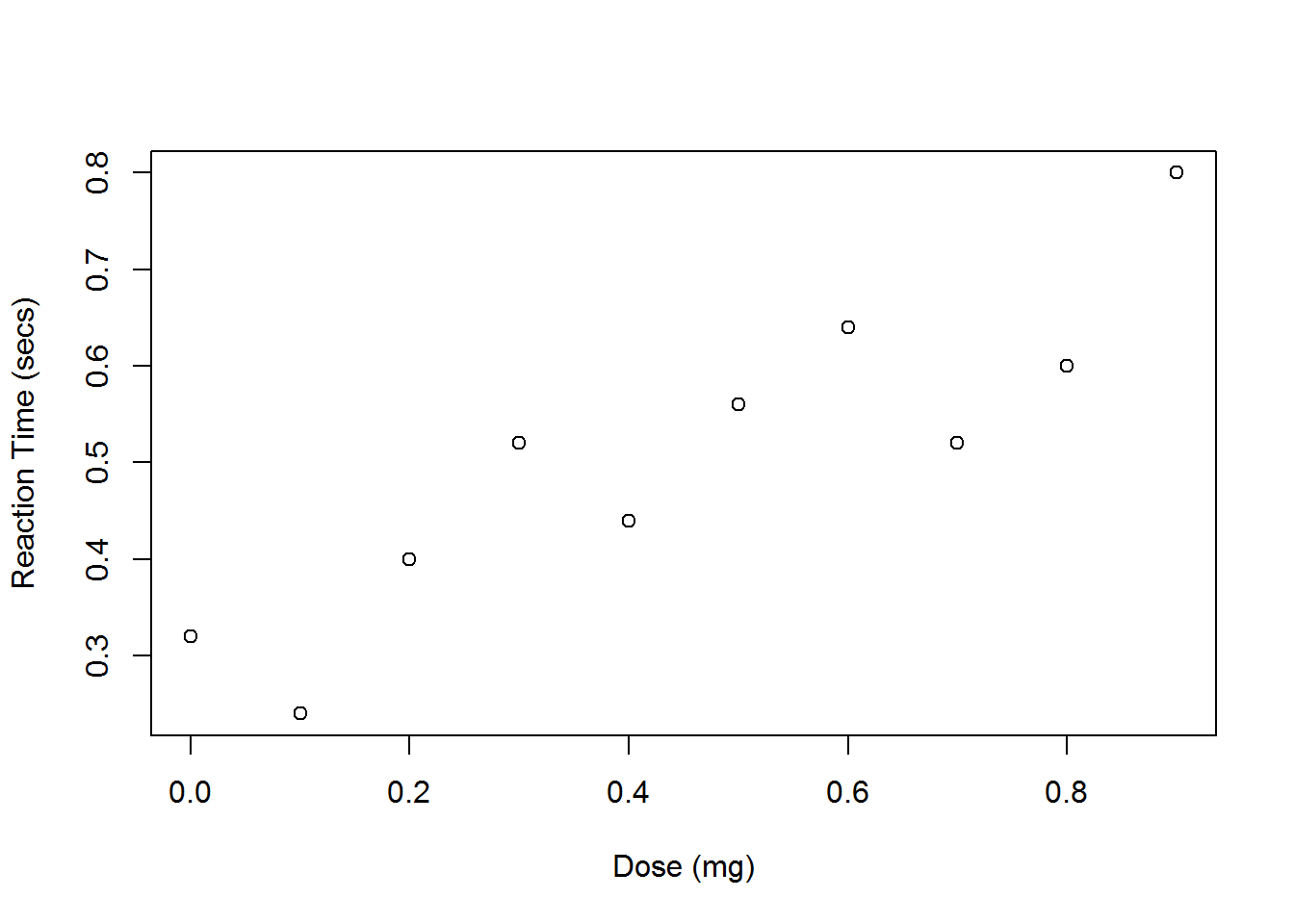A simple model for these data might be a straight line: $$E(y_i|x_i) = \beta_0 + \beta_1 x_i \text{ for } i = 1, \dots, n$$

The equivalent R command is given by:

The resulting regression line can be easy superposed to the data scatterplot by: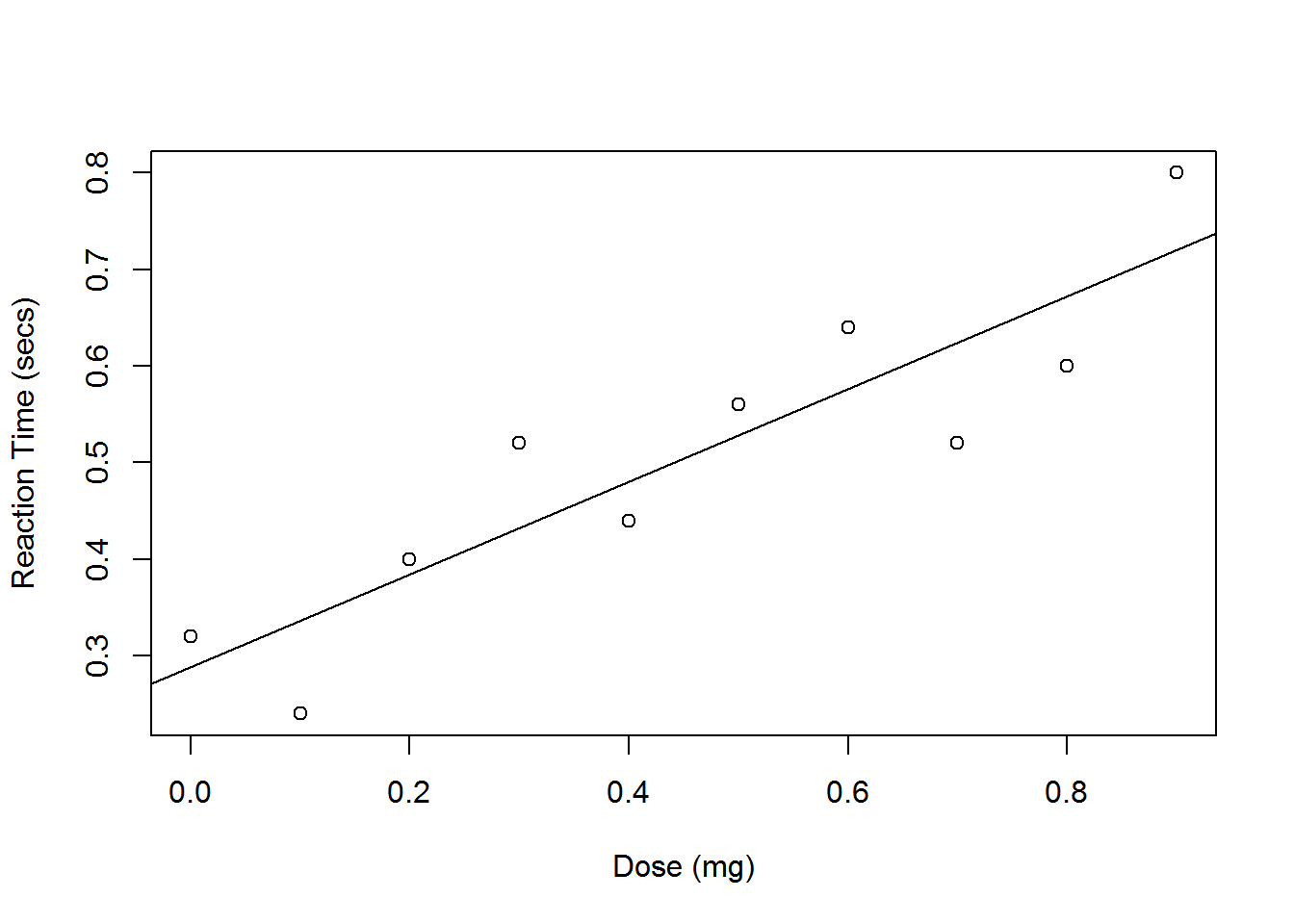Regression results can be investigated by generic function summary().

Small p-values for t-test on coefficients lead to consider both coefficients as significant. Adjust R-squared equal to 0.78 shows an acceptable portion of total variation explained by the regression model.

Small p-value for F-statistic confirm fitted model significance when compared with null model: $$E(y_i|x_i) = \beta_0$$.

Same result could be obtained by:

Prediction for response variable at specific values of the explanatories variable can be gained by:

Note how the newdata argument within predict() requires an object of class data.frame containing the same variables as in the explanatory part of the model.

Predict can be used for defining prediction interval for the fitted model. Prediction interval for any new observation possibly within the regression support is given by:

Prediction interval can be plotted by: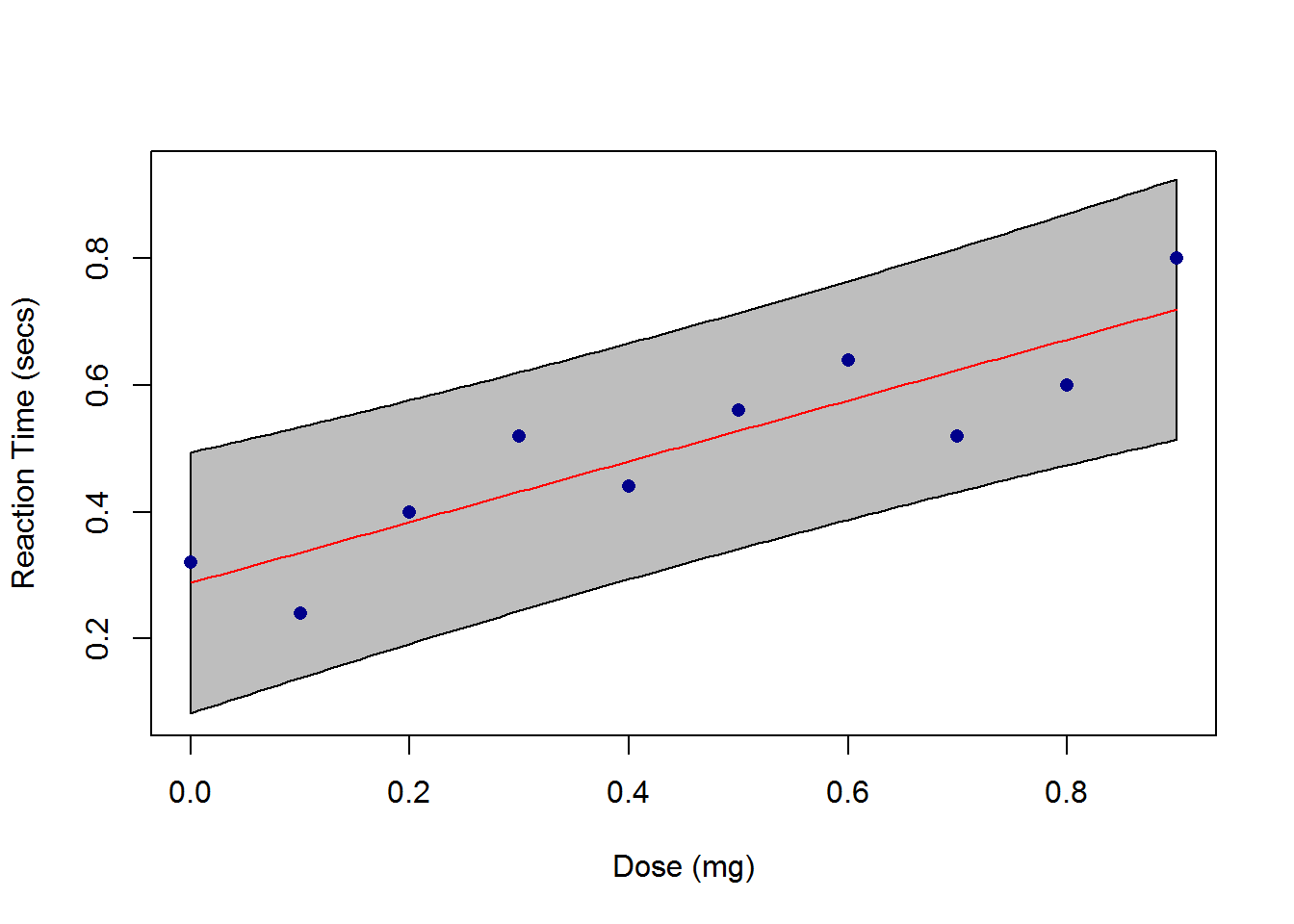Finally, the initial assumptions concerning the structure of the error term:

• $$E(\varepsilon_{i}) = 0$$ ($$i = 1, \dots, n$$)
• $$\varepsilon_i \sim\; N(0,\,\sigma^2_\varepsilon)$$ ($$i = 1, \dots, n$$)
• $$Corr(\varepsilon_{i},\,\varepsilon_{i-j}) = 0$$ ($$i = 1, \dots, n; 0 < j < i$$)

can be verified by the visual inspection of the suite of residuals plots offered by default by R: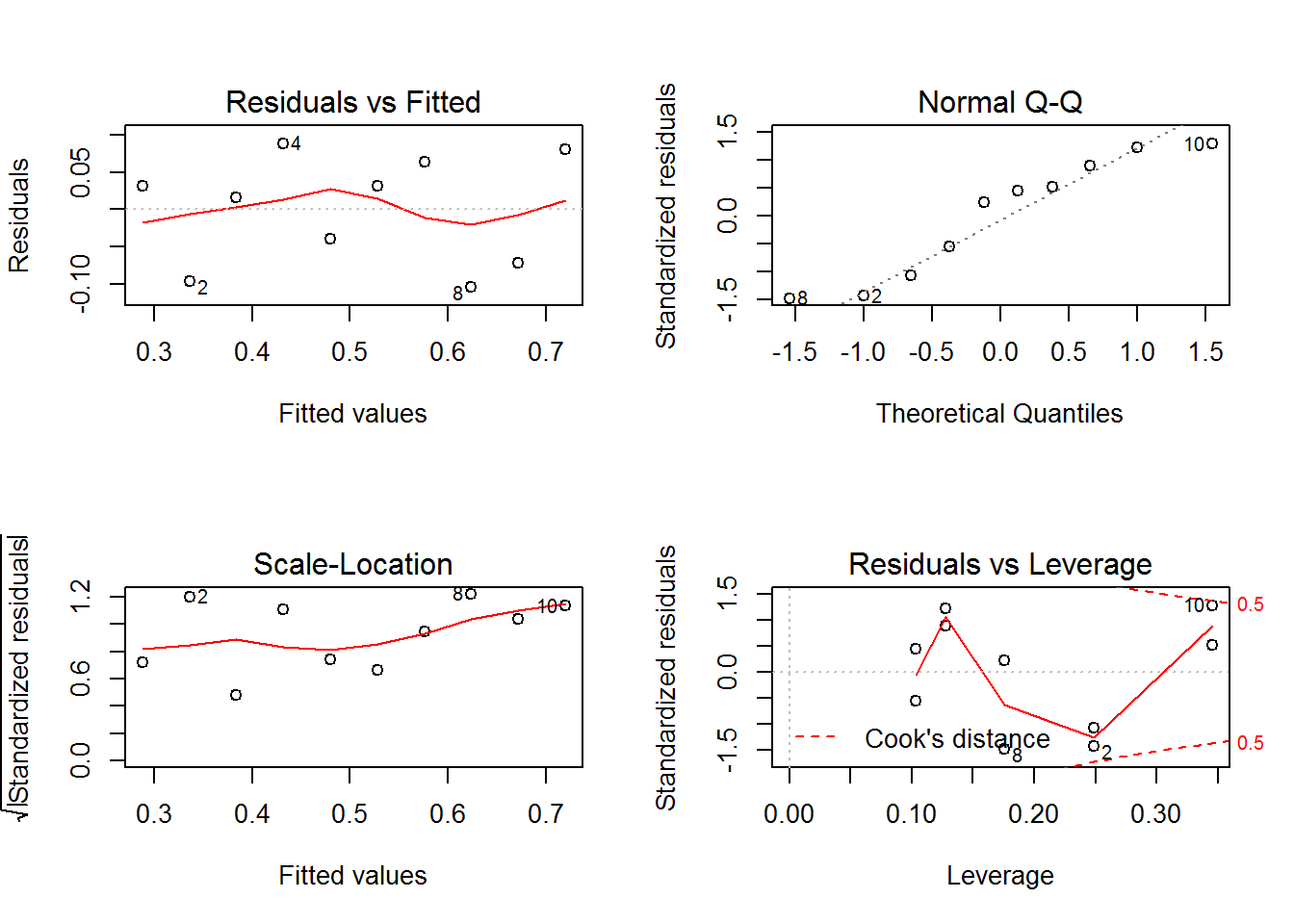The “Residuals vs Fitted” plot does not show, in this example, any particular pattern. The presence of patterns may suggest that the model is inadequate. The “Normal Q-Q” plot shows points close to the straight line. If the normal assumption of residuals is not satisfied, points are far from the straight line. The “Normal Q-Q plot” is less reliable on the distribution tails, i.e. points ought be very far from the straight line to suggest that residuals follow a non-normal distribution. The “Scale location” is similar to the residuals versus fitted values plot, but it uses the square root of the standardized residuals in order to diminish skewness. Like the first plot, there should be no discernable pattern to the plot. The “Residuals vs Leverage” shows leverage points. Leverage points are those observations, if any, made at extreme or outlying values of the independent variables such that the lack of neighboring observations means that the fitted regression model will pass close to that particular observation. Leverage points fall out the dotted lines.

### Car Seat

Three quality inspectors are studying the reproducibility of a measurement method aiming to test resistance of a specific material used to cover car seats. As a result, an experiments involving 75 samples of material from the same batch is set up. Three operators: Kevin, Michelle and Rob are assigned to test 25 samples each.

Comparison of operators average measurements and within operators variations are the key points of the analysis.

Boxplot of Strength by Operator along with within operators averages and between averages connecting line can be display by: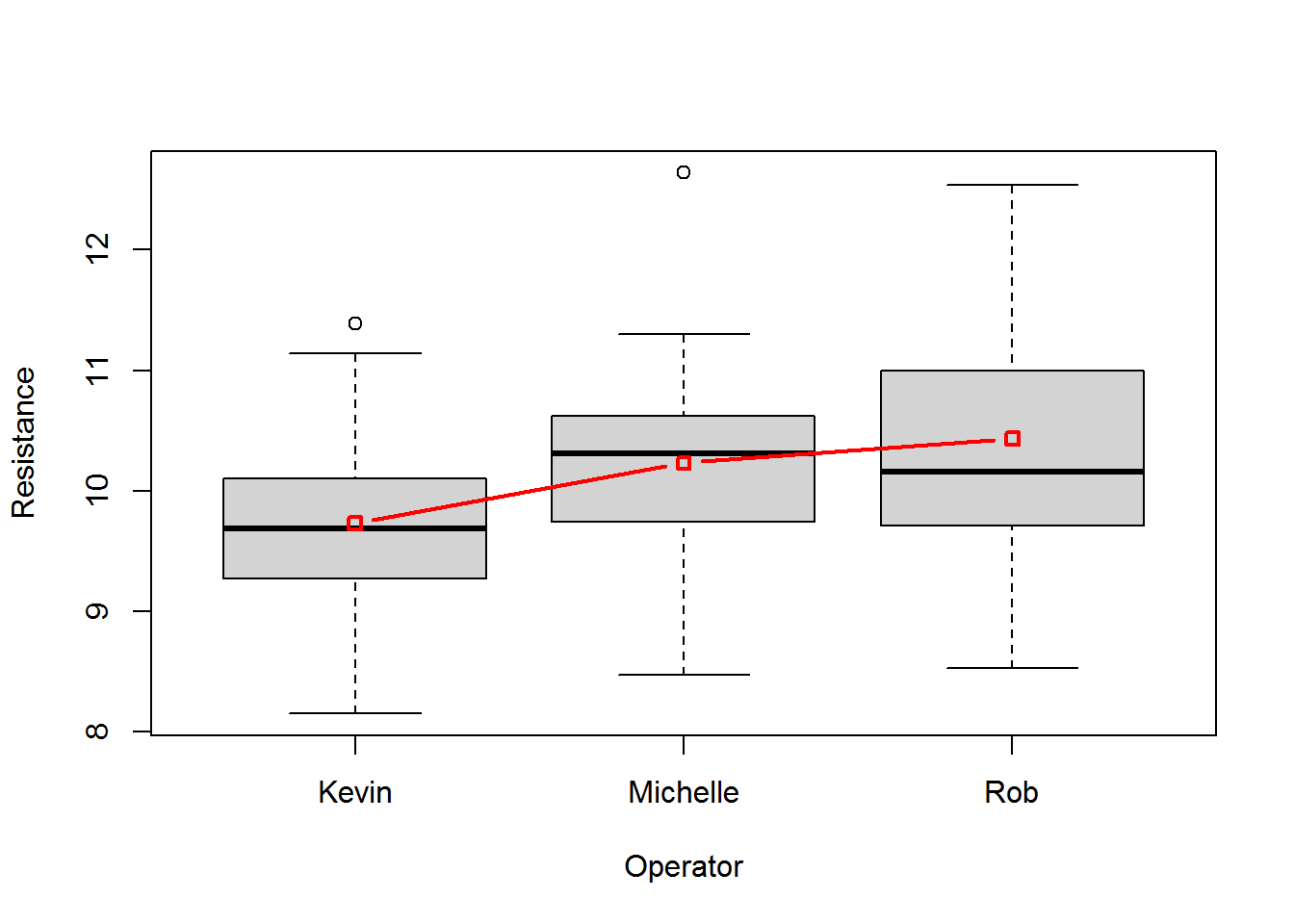At first glance, difference between operators is hard to detect as within variation seems to be quite large. An outstanding value is shown at Michelle.

A simple one way analysis of variance model is computed with:

Results are shown with the usual summary() method.

Operators appears to be borderline significant as its F test p-value is 0.0258. That is, not a real difference exists between operators measurements methods.

The overall influence of Michelle outstanding single result can be tested by repeating the analysis after the single observation is excluded from the data.

Operators significance is cleary increased as its p-value decreased to 0.0226. Nevertheless, practical results remain unchanged.

In mathematical notation the model can be written as: $$E(y_i|x_i) = \beta_0+\beta_j$$ with $$j = 1,2,3$$

Single coefficients can be visualized by:

Note that the intercept assumes the average strength value for Kevin while the other coefficients represent the difference in Strength between the corresponding operators and Kevin.

Finally, normality of residuals can be checked by: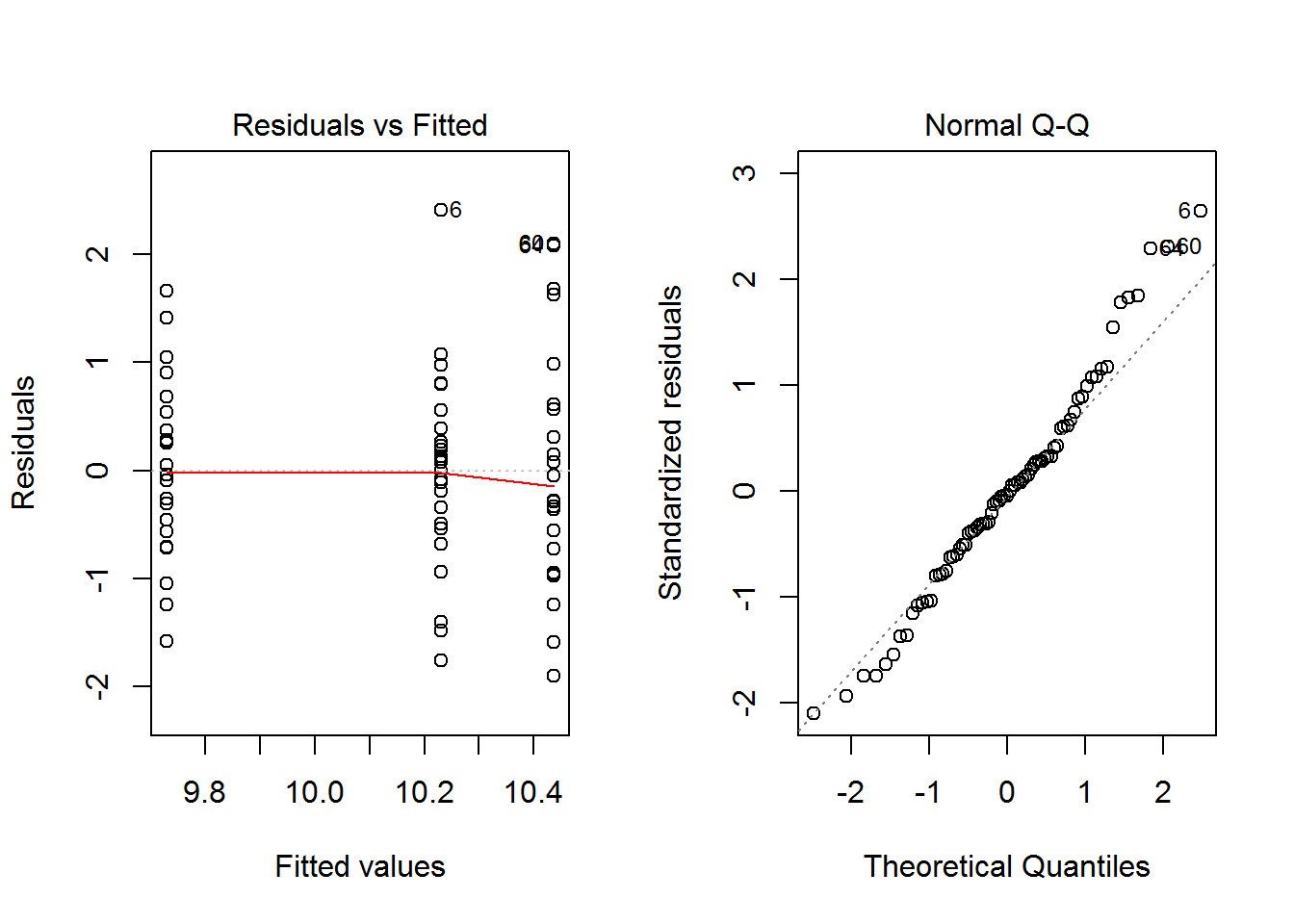Despite a small departure from normality on the rigth side of the residuals distribution, model assumptions seem to confirm.

### Boiling Time

In an attempt to resolve a domestic dispute about which of two pans was the quicker pan for cooking, the following data were obtained:

Various measured volumes (pints) of cold water were put into each pan and heated using the same setting of the cooker. The response variable was the time in minutes until the water boiled.

From a first visual investigation, it’s easy to understand that differences in boling time means difference either in intercept or slope between the two lines: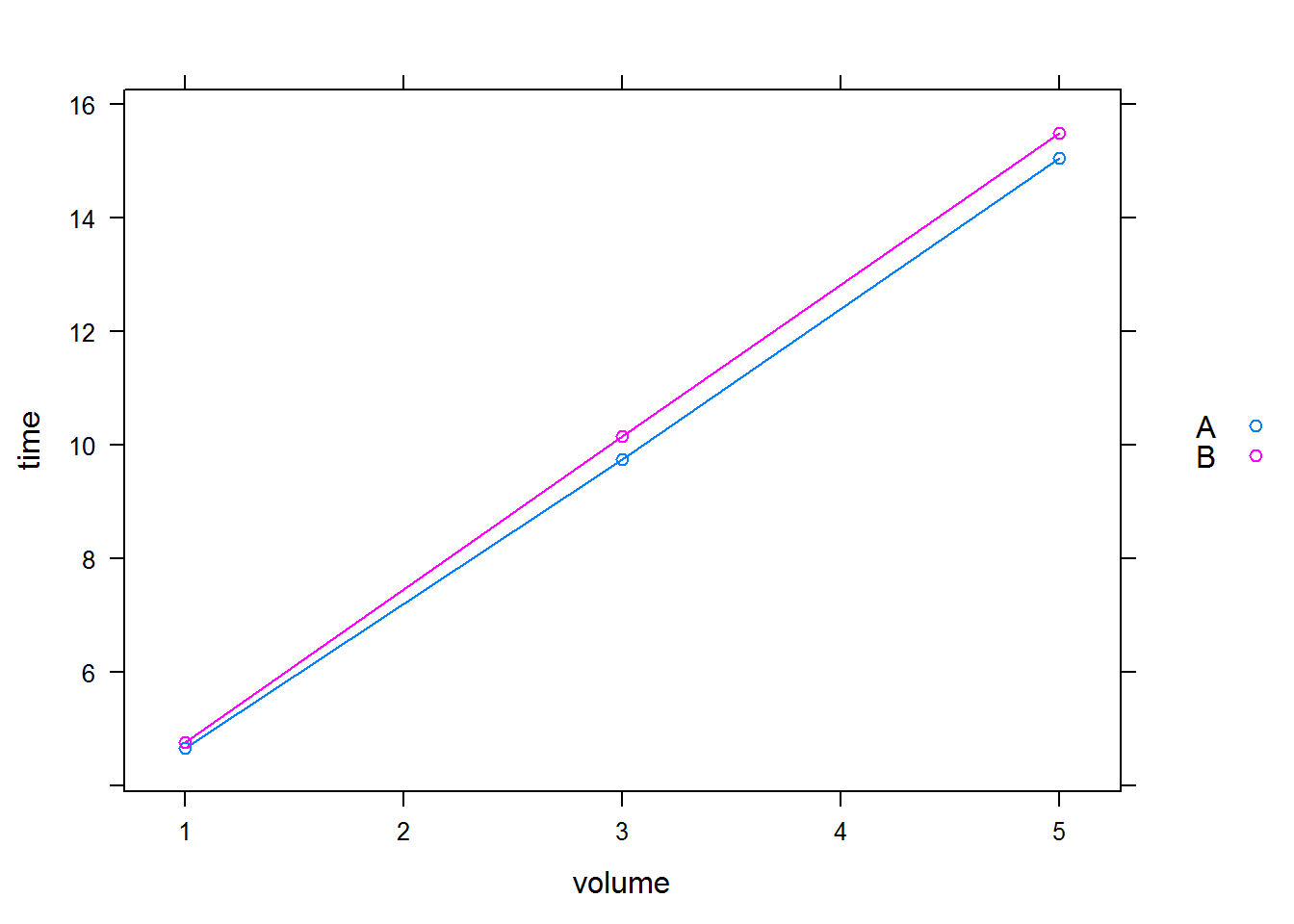A simple linear model may provide the answer to the above questions:

Given $$\alpha=0.05$$ as significance level, only some coefficients result as significant. Note that PanB express the difference between the intercept, aliased with PanA, and PanB while volume:panB represent the difference in slope between PanA (volume) and PanB.

PanA has a shorter boiling time than PanB but both the difference between the intercepts and the difference between slopes are not statistically significant.

A different parameterisation of the model, possibly more intuitive but less attinent with the original problem could be given by:

or alternatively by:

Finally, the same results could be obtained by using a generalized linear model with Gaussian family and its natural identity link function:

However, this model could be unsatisfactory in two respects:

• boiling time cannot be negative,
• the variance of boiling time might be expected to increase with its expectation.

The natural candidate for this problem is the gamma distibution as it respect the increasing relationship between mean and variance.

As a result, a generalized linear model with family gamma and link identity can be used in place of a standard liear model.

Models comparison can be given by the AIC statistics.

Gamma model appears to be slightly better.

## Summary

In this chapter, we introduced statistical modelling with R. Through some examples on real data, we saw the lm() function to build a linear regression model, the glm() function to build a generalised linear model and anova() and aov() functions to build an ANOVA model. The summary() function is useful to investigate regression and ANOVA results. We used the plot() function both to draw the regression line in the scatter plot and to perform a residual analysis. P value were used to choose between two nested models, while AIC statistics were introduced to compare two not-nested models.